Next: Enhancement factor of the Up: Beamstrahlung Previous: Algorithm of event generation

### Polarization

Polarization effects have been added since 2.1. In order to describe polarizations, let us introduce an orthonormal basis vector (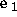,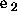,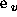). Here,is the unit vector along the initial electron velocity,the unit vector along the direction of the transverse component of acceleration, and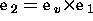. If the acceleration is due to a transverse magnetic field,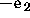is the unit vector along the magnetic field (times the sign of charge). The transition rate from the initial electron polarization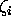to the final polarization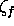with the photon Stokes parameter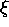(based on the basis vector (,,)) is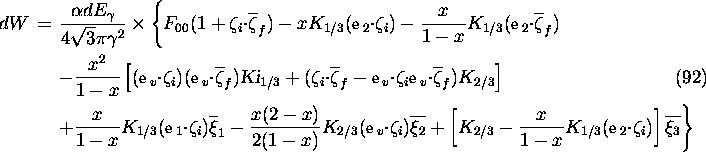where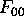is defined in eq.(85) and the argument of the Bessel functions is z. We omitted the terms involvingandsimultaneously, which means to ignore the correlation of polarization between the final electron and photon. (See Sec.5.2.2 for the meaning of bars onand.)

The radiation energy spectrum summed over the final polarization is given by eq.(85) withreplaced by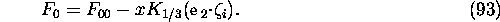Since the function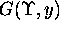withreplaced by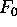has still the above mentioned property, the same algorithm of generating the photon energy can be used. (is slightly larger when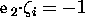but still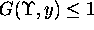.)

For the given radiation energy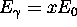, the polarizations of the final electron and photon are calculated by the prescription described in Sec.5.2.2. Thus,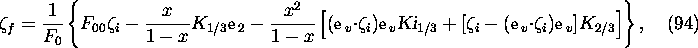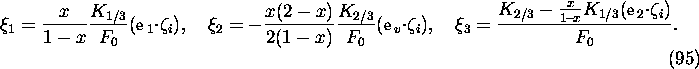In the case when the event generation is rejected, the polarization of the electron must be changed according to eq.(12):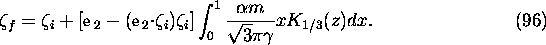In storage rings, the electron polarization builds up slowly along the direction of the magnetic field. This effect comes from the difference between the coefficient ofand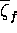(see eq.(14)):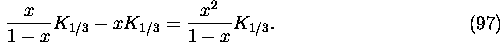When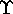is small (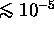in storage rings), each term on the left hand side is proportional towhereas the right hand side is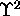because of cancellation. CAIN cannot reproduce such slow buildup, even if the computing time allows, because the approximate polynomials adopted do not have that accuracy. They are enough, however, for beam-beam problems.Next: Enhancement factor of the Up: Beamstrahlung Previous: Algorithm of event generation

Toshiaki Tauchi
Thu Dec 3 17:27:26 JST 1998